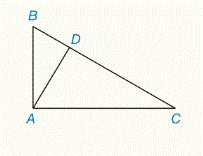Chapter 5.CR, Problem 30CRElementary Geometry For College St...

7th Edition
Alexander + 2 others
ISBN: 9781337614085

Solutions

Chapter
SectionElementary Geometry For College St...

7th Edition
Alexander + 2 others
ISBN: 9781337614085
Textbook Problem

Given: Δ A B C       with     right     ∠ B A C A D ¯ ⊥ B C ¯ a ) B D = 3 , A D = 5 , D C = ? b ) A C = 10 , D C = 4 , B D = ? c ) B D = 2 , B C = 6 , B A = ? d ) B D = 3 , A C = 3 2 , D C = ?To determine

a)

To find:

DC

Explanation

Given:

ΔABC with right BACAD¯BC¯

And

That is,

Approach:

By using angle-bisector theorem, that is “if a ray bisects of an angle of a triangle, then it divides the opposite side into two segments whose length are proportional to the lengths of the two sides that from the bisected angle”

Let x be the length of DC

To determine

b)

To find:

The value of BD.

To determine

c)

To find:

BA

To determine

d)

To find:

The value of DC.

Still sussing out bartleby?

Check out a sample textbook solution.

See a sample solution

The Solution to Your Study Problems

Bartleby provides explanations to thousands of textbook problems written by our experts, many with advanced degrees!

Get Started

Find more solutions based on key concepts